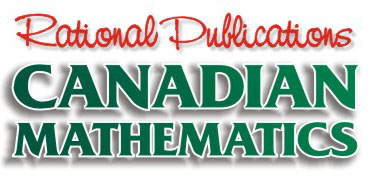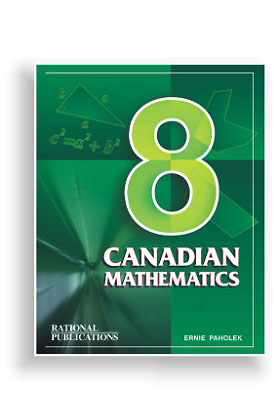The following summary contains a complete listing of the chapters, along with a sample question from each chapter.

Should you have further questions about the text book, please contact us.

If you wish to order Canadian Mathematics 8 or any of the other Rational Publications text books, please do so by clicking on the order form.

 CHAPTER 1 - WHOLE NUMBERS 1.1 Place Value of Whole Numbers 1.2 Graphing Whole Numbers 1.3 Rounding & Estimation 1.4 Addition & Subtraction 1.5 Multiplication & Division 1.6 Order of Operations (BEDMAS) 1.7 Substitution 1.8 Properties of Whole Numbers 1.9 Review CHAPTER 2 - FACTORS & MULTIPLES 2.1 Rules for Divisibility 2.2 Factors 2.3 Multiples 2.4 Prime & Composite Numbers 2.5 Prime Factorization 2.6 Greatest Common Factor (GCF) 2.7 Lowest Common Multiple (LCM) 2.8 Review CHAPTER 3 - FRACTIONS & DECIMALS 3.1 Equivalent & Basic Fractions 3.2 Addition & Subtraction 3.3 Multiplication & Division 3.4 Review 3.5 Decimal Notation 3.6 Converting Decimals <—> Fractions 3.7 Computation with Decimals CHAPTER 4 - INTEGERS 4.1 Positive & Negative Integers 4.2 Addition of Integers 4.3 Subtraction of Integers 4.4 Addition & Subtraction 4.5 Multiplication of Integers 4.6 Division of Integers 4.7 Multiplication & Division 4.8 Order of Operations (BEDMAS) 4.9 Plotting Integers 4.10 Functions & Function Tables 4.11 Review CHAPTER 5 - RATIONAL NUMBERS 5.1 Computation with Rationals 5.2 Order of Rational Numbers 5.3 Addition & Subtraction 5.4 Multiplication & Division 5.5 Review 5.6 Order of Operations (BEDMAS) 5.7 Substitution 5.8 Review CHAPTER 6 - EQUATIONS AND INEQUALITIES 6.1 Solving Type I Equations 6.2 Solving Type II Equations 6.3 Solving Type III Equations 6.4 Solving Type IV Equations 6.5 Solving Ratio Equations 6.6 Equations Review 6.7 Inequalities & Graphing 6.8 Review CHAPTER 7 - PROBLEM SOLVING USING EQUATIONS 7.1 Mathematics <—> English 7.2 Solving Number Problems 7.3 Solving Geometric Problems 7.4 Number & Geometric Review 7.5 Age Problems 7.6 Coin Problems 7.7 Problems Solving Review 7.8 Equation & Problems Review CHAPTER 8 - RATE, RATIO & PERCENT 8.1 Ratio 8.2 Ratio, Proportion & Rate 8.3 Percent Problems 8.4 Discount Problems 8.5 Commission Problems 8.6 Calculating Sales Tax 8.7 Interest Problems 8.8 Percent Problems 8.9 Review CHAPTER 9 - MEASUREMENT (METRIC & ANGULAR) 9.1 Linear Measurement 9.2 Linear Conversions 9.3 Area Measurements 9.4 Area Conversion 9.5 Volume Measurements 9.6 Volume Conversions 9.7 Linear, Area & Volume 9.8 Volume, Capacity & Mass 9.9 Angular Measurements 9.10 Review CHAPTER 10 - GEOMETRY 10.1 Motion Geometry (The Slide) 10.2 Motion Geometry (The Turn) 10.3 Motion Geometry (The Flip) 10.4 Similar Figures 10.5 Angles 10.6 Polygons 10.7 Triangles 10.8 Quadrilaterals 10.9 Interior Angle Sum (Polygons) 10.10 Congruency of Triangles 10.11 Similar Triangles 10.12 Review CHAPTER 11 - PERIMETER, AREA & VOLUME 11.1 Perimeter of Polygons 11.2 Perimeter (Quadrilaterals) 11.3 Perimeter (Triangles & Polygons) 11.4 Area (Quadrilaterals) 11.5 Area (Triangles & Trapezoids) 11.6 Problems (Squares & Rectangles) 11.7 Problems (Parallelograms) 11.8 Problems (Quadrilaterals) 11.9 Problems (Triangles) 11.10 Problems (Trapezoids) 11.11 Circles (Circumference & Area) 11.12 Area of Shaded Regions 11.13 Volume of Prisms 11.14 Review CHAPTER 12 - PYTHAGOREAN THEOREM 12.1 Triangles - vertices & sides 12.2 Right Angle Triangles 12.3 Area Using Pythagoras 12.4 Pythagorean Triplets & Triads 12.5 Square Roots 12.6 Pythagorean Theorem Calculations 12.7 Review CHAPTER 13 - EXPONENTS 13.1 Positive Exponents 13.2 Negative Exponents 13.3 Multiplication With Exponents 13.4 Division With Exponents 13.5 Power of a Power Property 13.6 Computation With Exponents 13.7 Scientific Notation 13.8 Review CHAPTER 14 - DATA MANAGEMENT 14.1 Statistics 14.2 Mode,Median,Range, Mean 14.3 Box and Whisker Plot 14.4 Stem and Leaf Plot 14.5 Broken Line Graphs 14.6 Bar Graphs 14.7 Circle Graphs 14.8 Probability 14.9 Independent Events 14.10 Review

Home   |   Math 7   |   Math 9    |   Order Form   |   Contact Us